Excel VBA Comparison Operators

• Last Updated : 05 Jul, 2021

VBA in Excel stands for Visual Basic for Applications which is Microsoft’s programming language. To optimize the performance and reduce the time in Excel we need Macros and VBA is the tool used in the backend.

In this article, we are going to discuss various comparison operators in Excel VBA.

Implementation :

In the Microsoft Excel tabs, select the Developer Tab. Initially, the Developer Tab may not be available.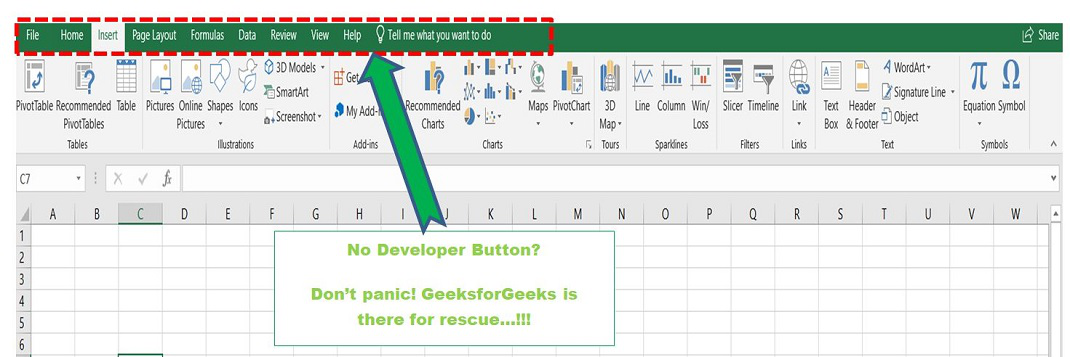The Developer Tab can be enabled easily by a two-step process :

• Right-click on any of the existing tabs in the top of the Excel window.
• Now select Customize the Ribbon from the pop-down menu.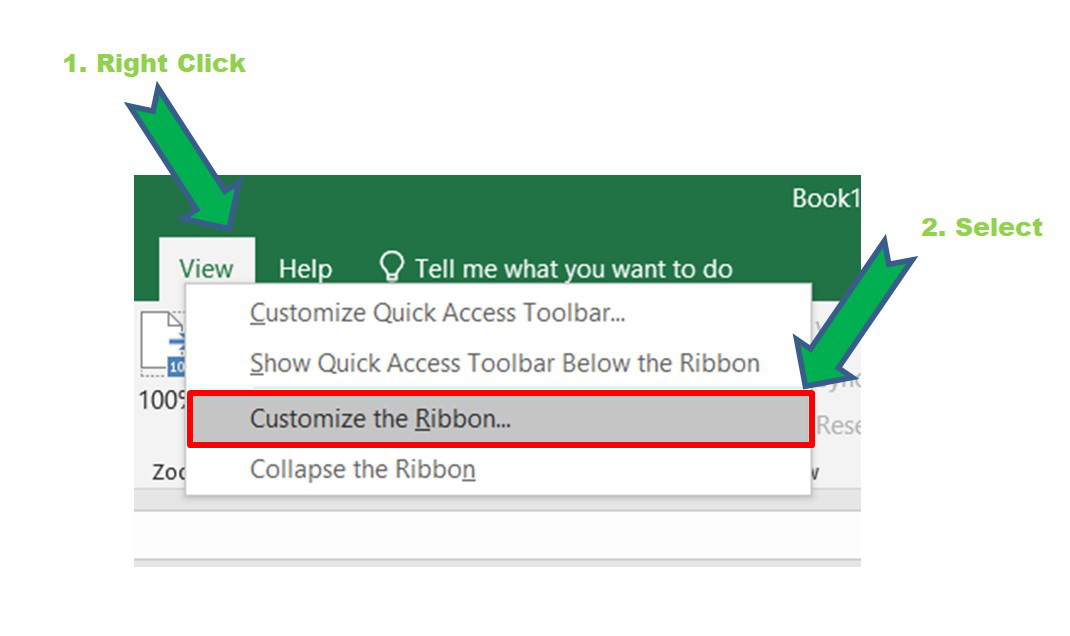• The Excel Options Box, check the box Developer to enable it and click on OK.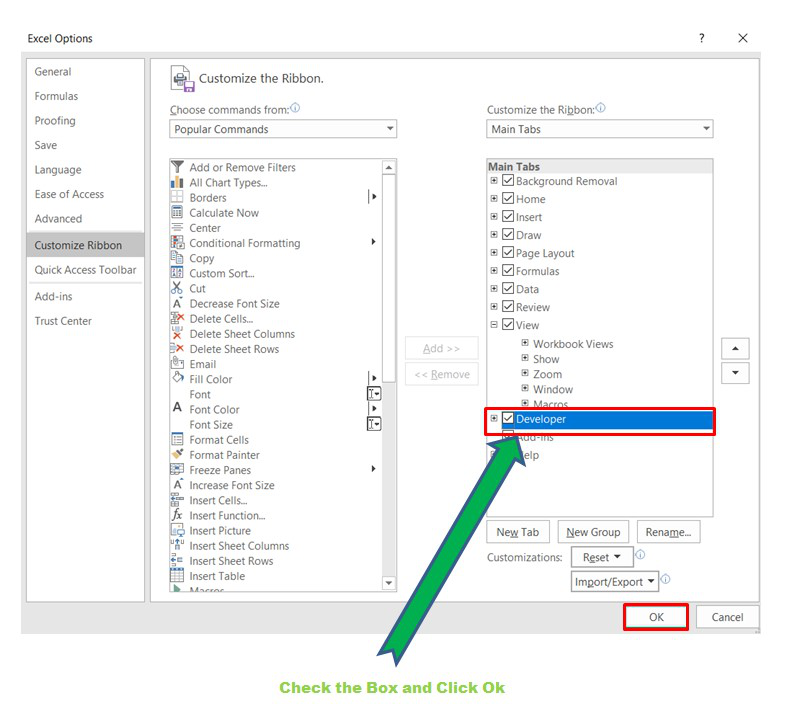• Now, the Developer Tab is visible.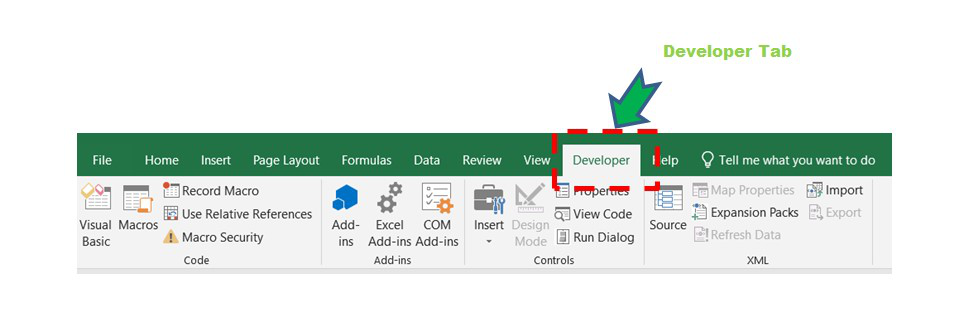• Now click on the Visual Basic option in the Developer tab and make a new module to write the program.
Developer  -> Visual Basic -> Tools -> Macros

Now create a Macro and give any suitable name.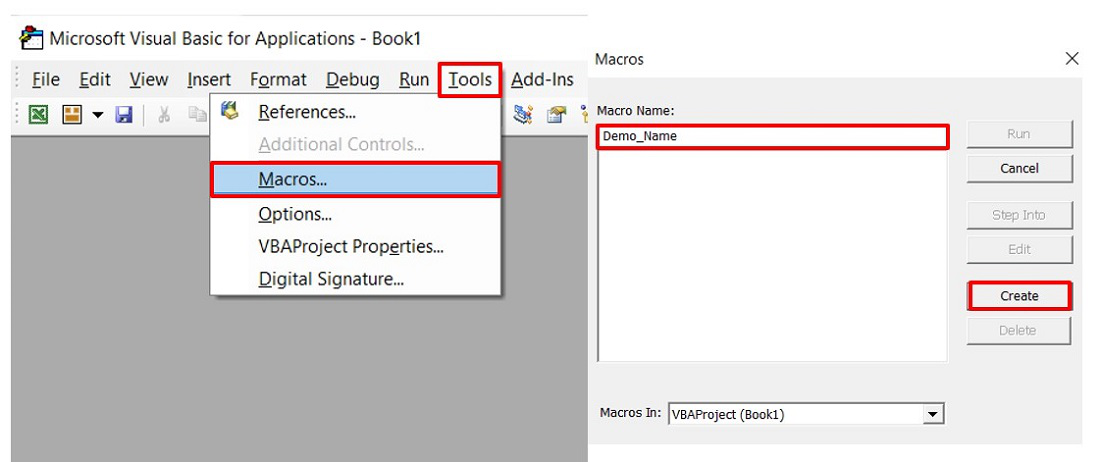This will open the Editor window where can write the code.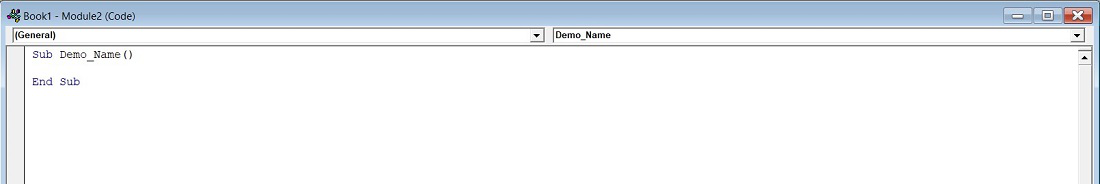Comparison Operators in Excel:

The comparison operators are mostly used with the If Else Then statement in Excel because comparison operators return TRUE if the condition is met and FALSE if not.

The syntax of If Else in Excel is :

If condition/expression Then
Code Block for True
Else
Code Block for False
End If

Let’s take an example where values of A=-1 and B=-5 and see the code in Excel VBA for all the comparison operators.

1. Equal To and Not Equal To

Sub Comparison_Operator_Demo()
'Entering the numbers
Dim A As Integer: A = -1
Dim B As Integer: B = -5
'Condition for Equal To
If A = B Then
MsgBox " A and B are equal"
Else
MsgBox " A and B are not equal"
End If
End Sub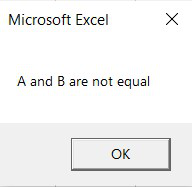In the above code, If the condition becomes FALSE since A and B values are not the same. So the code block inside Else will be executed.

Sub Comparison_Operator_Demo()
'Entering the numbers
Dim A As Integer: A = -1
Dim B As Integer: B = -5
'Condition for Not Equal To
If A <> B Then
MsgBox " True since A and B are not same"
Else
MsgBox " False since A and B are same"
End If
End Sub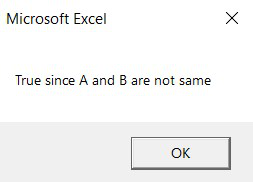In the above code If the condition becomes TRUE since A and B are not same. So the code block inside If will be executed.

2. Greater than or Less than operator :

Sub Comparison_Operator_Demo()
'Entering the numbers
Dim A As Integer: A = -1
Dim B As Integer: B = -5
'Condition for Greater Than
If A > B Then
MsgBox " A is greater than B"
Else
MsgBox " A is not greater than B"
End If
End Sub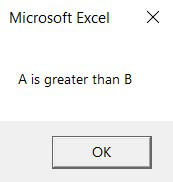In the above code If the condition becomes TRUE since A is greater than B. So the code block inside If will be executed.

Sub Comparison_Operator_Demo()
'Entering the numbers
Dim A As Integer: A = -1
Dim B As Integer: B = -5
'Condition for Less Than
If A < B Then
MsgBox "True because A is less than B"
Else
MsgBox "False because A is greater than B"
End If
End Sub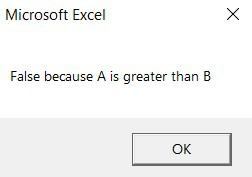In the above code If the condition becomes FALSE since A is greater than B. So the code block inside Else will be executed.

Similarly, you can do for Greater than equal to and Less than equal to operators.

My Personal Notes arrow_drop_up